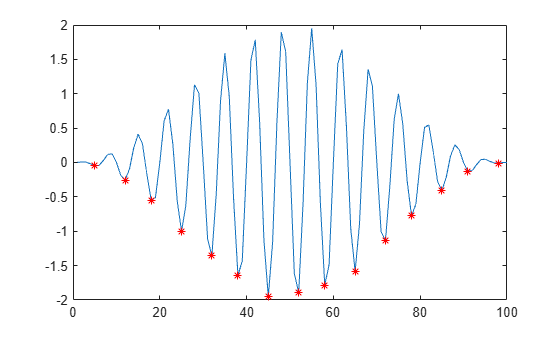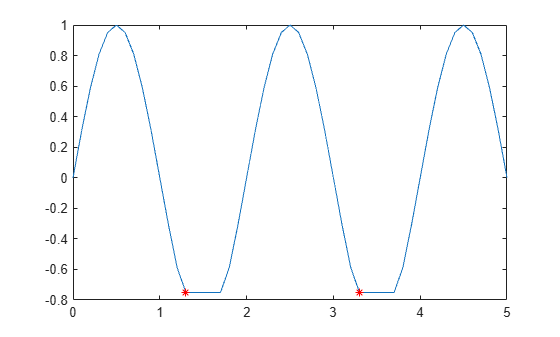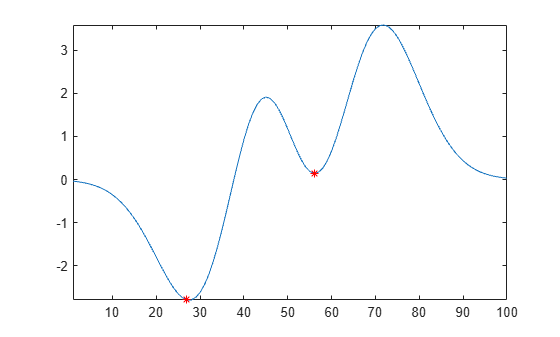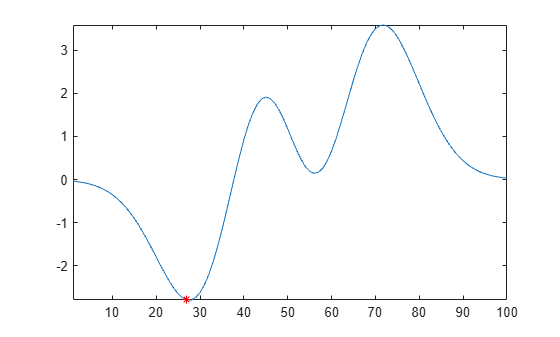# islocalmin

Find local minima

## Syntax

``TF = islocalmin(A)``
``TF = islocalmin(A,dim)``
``TF = islocalmin(___,Name,Value)``
``[TF,P] = islocalmin(___)``

## Description

example

````TF = islocalmin(A)` returns a logical array whose elements are 1 (`true`) when a local minimum is detected in the corresponding element of `A`.```

example

````TF = islocalmin(A,dim)` specifies the dimension of `A` to operate along. For example, `islocalmin(A,2)` finds the local minimum of each row of a matrix `A`.```

example

````TF = islocalmin(___,Name,Value)` specifies parameters in addition to any of the input argument combinations in previous syntaxes for finding local minima using one or more name-value arguments. For example, `islocalmin(A,'SamplePoints',t)` finds local minima of `A` with respect to the time stamps contained in the time vector `t`.```

example

````[TF,P] = islocalmin(___)` also returns the prominence corresponding to each element of `A`.```

## Examples

collapse all

Compute and plot the local minima of a vector of data.

```x = 1:100; A = (1-cos(2*pi*0.01*x)).*sin(2*pi*0.15*x); TF = islocalmin(A); plot(x,A,x(TF),A(TF),'r*')```Create a matrix of data, and compute the local minima for each row.

```A = -25*diag(ones(5,1)) + rand(5,5); TF = islocalmin(A,2)```
```TF = 5x5 logical array 0 0 0 1 0 0 1 0 0 0 0 0 1 0 0 0 0 0 1 0 0 0 1 0 0 ```

Compute the local minima of a vector of data relative to the time stamps in the vector `t`. Use the `MinSeparation` parameter to compute minima that are at least 45 minutes apart.

```t = hours(linspace(0,3,15)); A = [2 4 6 4 3 7 5 6 5 10 4 -1 -3 -2 0]; TF = islocalmin(A,'MinSeparation',minutes(45),'SamplePoints',t); plot(t,A,t(TF),A(TF),'r*')```Specify a method for indicating consecutive minima values.

Compute the local minima of data that contains consecutive minima values. Indicate the minimum of each flat region based on the first occurrence of that value.

```x = 0:0.1:5; A = max(-0.75, sin(pi*x)); TF1 = islocalmin(A,'FlatSelection','first'); plot(x,A,x(TF1),A(TF1),'r*')```Indicate the minimum of each flat region with all occurrences of that value.

```TF2 = islocalmin(A,'FlatSelection','all'); plot(x,A,x(TF2),A(TF2),'r*')```Compute the local minima of a vector of data and their prominence, and then plot them with the data.

```x = 1:100; A = peaks(100); A = A(50,:); [TF1,P] = islocalmin(A); P(TF1)```
```ans = 1×2 2.7585 1.7703 ```
```plot(x,A,x(TF1),A(TF1),'r*') axis tight```Compute the most prominent minimum in the data by specifying a minimum prominence requirement.

```TF2 = islocalmin(A,'MinProminence',2); plot(x,A,x(TF2),A(TF2),'r*') axis tight```## Input Arguments

collapse all

Input data, specified as a vector, matrix, multidimensional array, table, or timetable.

Operating dimension, specified as a positive integer scalar. If no value is specified, then the default is the first array dimension whose size does not equal 1.

Consider an `m`-by-`n` input matrix, `A`:

• `islocalmin(A,1)` computes local minima according to the data in each column of `A` and returns an `m`-by-`n` matrix.• `islocalmin(A,2)` computes local minima according to the data in each row of `A` and returns an `m`-by-`n` matrix.For table or timetable input data, `dim` is not supported and operation is along each table or timetable variable separately.

### Name-Value Arguments

Specify optional pairs of arguments as `Name1=Value1,...,NameN=ValueN`, where `Name` is the argument name and `Value` is the corresponding value. Name-value arguments must appear after other arguments, but the order of the pairs does not matter.

Before R2021a, use commas to separate each name and value, and enclose `Name` in quotes.

Example: `TF = islocalmin(A,'MinProminence',2)`

Data Options

collapse all

Sample points, specified as a vector of sample point values or one of the options in the following table when the input data is a table. The sample points represent the x-axis locations of the data, and must be sorted and contain unique elements. Sample points do not need to be uniformly sampled. The vector ```[1 2 3 ...]``` is the default.

When the input data is a table, you can specify the sample points as a table variable using one of these options.

Option for Table InputDescriptionExamples
Variable name

A character vector or scalar string specifying a single table variable name

`'Var1'`

`"Var1"`

Scalar variable index

A scalar table variable index

`3`

Logical vector

A logical vector whose elements each correspond to a table variable, where `true` specifies the corresponding variable as the sample points, and all other elements are `false`

`[true false false]`

Function handle

A function handle that takes a table variable as input and returns a logical scalar, which must be `true` for only one table variable

`@isnumeric`

`vartype` subscript

A table subscript generated by the `vartype` function that returns a subscript for only one variable

`vartype('numeric')`

Note

This name-value argument is not supported when the input data is a `timetable`. Timetables always use the vector of row times as the sample points. To use different sample points, you must edit the timetable so that the row times contain the desired sample points.

Example: `islocalmin(A,'SamplePoints',0:0.1:10)`

Example: `islocalmin(T,'SamplePoints',"Var1")`

Table variables to operate on, specified as the comma-separated pair consisting of `DataVariables` and one of the options in this table. The `DataVariables` value indicates which variables of the input table to examine for local minima. The data type associated with the indicated variables must be numeric or `logical`.

The first output `TF` contains `false` for variables not specified by `DataVariables` unless the value of `OutputFormat` is `'tabular'`.

OptionDescriptionExamples
Variable name

A character vector or scalar string specifying a single table variable name

`'Var1'`

`"Var1"`

Vector of variable names

A cell array of character vectors or string array where each element is a table variable name

`{'Var1' 'Var2'}`

`["Var1" "Var2"]`

Scalar or vector of variable indices

A scalar or vector of table variable indices

`1`

`[1 3 5]`

Logical vector

A logical vector whose elements each correspond to a table variable, where `true` includes the corresponding variable and `false` excludes it

`[true false true]`

Function handle

A function handle that takes a table variable as input and returns a logical scalar

`@isnumeric`

`vartype` subscript

A table subscript generated by the `vartype` function

`vartype('numeric')`

Example: ```islocalmin(T,'DataVariables',["Var1" "Var2" "Var4"])```

Output data type, specified as one of these values:

• `'logical'` — For table or timetable input data, return the output `TF` as a logical array.

• `'tabular'` — For table input data, return the output `TF` as a table. For timetable input data, return the output `TF` as a timetable.

For vector, matrix, or multidimensional array input data, `OutputFormat` is not supported.

Example: `islocalmin(T,'OutputFormat','tabular')`

Extrema Detection Options

collapse all

Minimum prominence, specified as a nonnegative scalar. `islocalmin` returns only local minima whose prominence is at least the value specified.

Prominence window, specified as a positive integer scalar, a two-element vector of positive integers, a positive duration scalar, or a two-element vector of positive durations. The value defines a window of neighboring points for which to compute the prominence for each local minimum.

When the window value is a positive integer scalar k, then the window is centered about each local minimum and contains `k-1` neighboring elements. If k is even, then the window is centered about the current and previous elements. If a local minimum is within a flat region, then `islocalmin` treats the entire flat region as the center point of the window.

When the value is a two-element vector of positive integers `[b f]`, then the window contains the local minimum, `b` elements backward, and `f` elements forward. If a local minimum is within a flat region, then the window starts `b` elements before the first point of the region and ends `f` elements after the last point of the region.

When the input data is a timetable or `SamplePoints` is specified as a `datetime` or `duration` vector, the window value must be of type `duration`, and the window is computed relative to the sample points.

Flat region indicator for when a local minimum value is repeated consecutively, specified as one of these values:

• `'center'` — Indicate only the center element of a flat region as the local minimum. The element of `TF` corresponding to the center of the flat is 1, and is 0 for the remaining flat elements.

• `'first'` — Indicate only the first element of a flat region as the local minimum. The element of `TF` corresponding to the start of the flat is 1, and is 0 for the remaining flat elements.

• `'last'` — Indicate only the last element of a flat region as the local minimum. The element of `TF` corresponding to the end of the flat is 1, and is 0 for the remaining flat elements.

• `'all'` — Indicate all the elements of a flat region as the local minima. The elements of `TF` corresponding to all parts of the flat are 1.

When using the `MinSeparation` or `MaxNumExtrema` name-value arguments, flat region points are jointly considered a single minimum point.

Minimum separation between local minima, specified as a nonnegative scalar. The separation value is defined in the same units as the sample points vector, which is `[1 2 3 ...]` by default. When the separation value is greater than 0, `islocalmin` selects the smallest local minimum and ignores all other local minima within the specified separation. This process is repeated until there are no more local minima detected.

When the sample points vector has type `datetime`, the separation value must have type `duration`.

Maximum number of minima to detect, specified as a positive integer scalar. `islocalmin` finds no more than the specified number of the most prominent minima, which is the length of the operating dimension by default.

## Output Arguments

collapse all

Local minima indicator, returned as a vector, matrix, multidimensional array, table, or timetable.

`TF` is the same size as `A` unless the value of `OutputFormat` is `'tabular'`. If the value of `OutputFormat` is `'tabular'`, then `TF` only has variables corresponding to the `DataVariables` specified.

Data Types: `logical`

Prominence, returned as a vector, matrix, multidimensional array, table, or timetable.

• If `P` is a vector, matrix, or multidimensional array, `P` is the same size as `A`.

• If `P` is a table or timetable, `P` is the same height as `A` and only has variables corresponding to the `DataVariables` specified.

If the input data has a signed or unsigned integer type, then `P` is an unsigned integer.

collapse all

### Prominence of Local Minimum

The prominence of a local minimum (or valley) measures how the valley stands out with respect to its depth and location relative to other valleys.

To measure the prominence of a valley, first extend a horizontal line from the valley. Find where the line intersects the data on the left and on the right, which will either be another valley or the end of the data. Mark these locations as the outer endpoints of the left and right intervals. Next, find the highest peak in both the left and right intervals. Take the smaller of these two peaks, and measure the vertical distance from that peak to the valley. This distance is the prominence.

For a vector `x`, the largest prominence is at most `max(x)-min(x)`.

## Version History

Introduced in R2017b

expand all